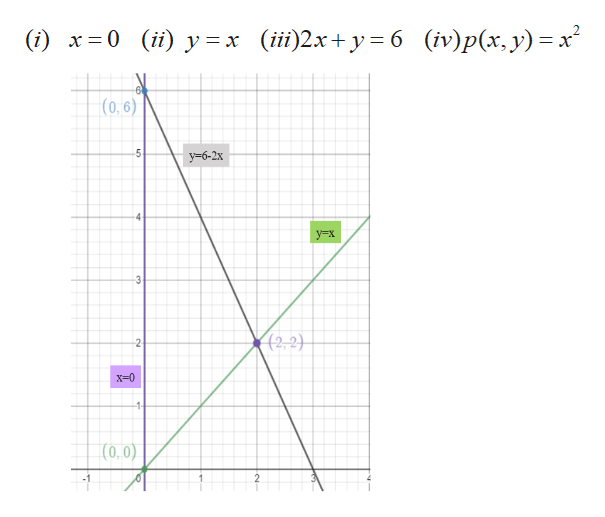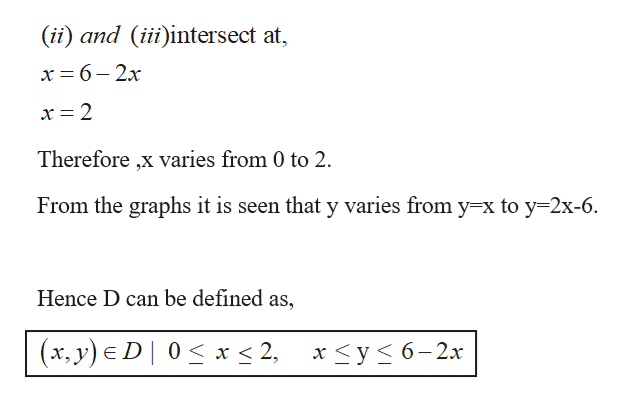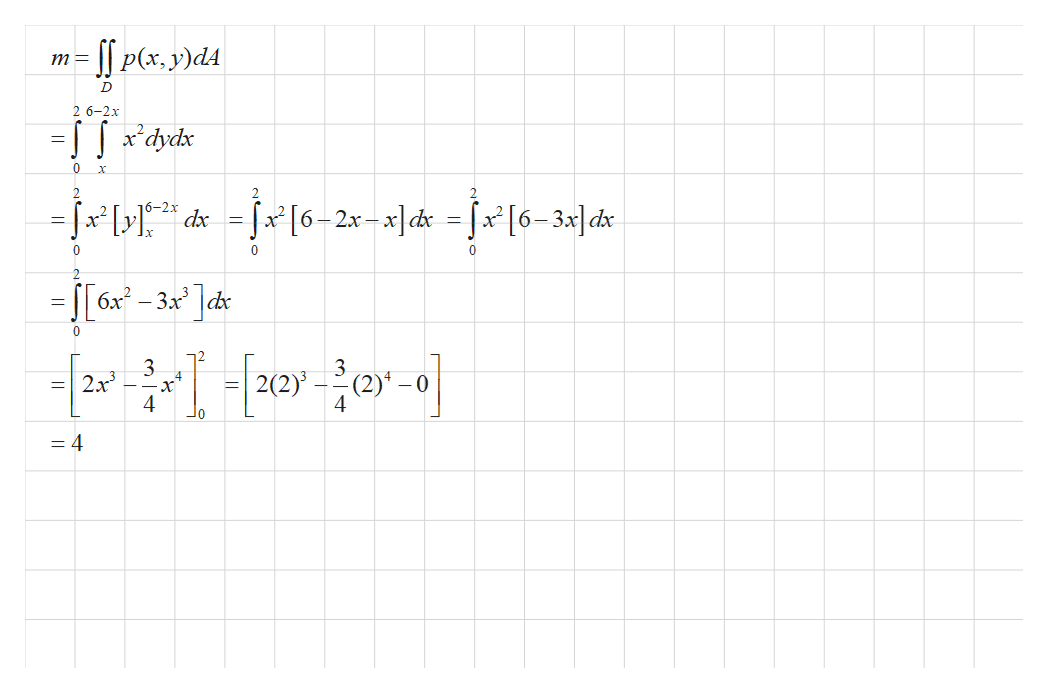# Find the mass and center of mass of the lamina that occupies the region D and has the given density function p. D is the triangular region enclosed by the lines x=0, y=x, and 2x+y=6; p(x,y)=x^2. Can you solve this problem with steps?

Question
108 views

Find the mass and center of mass of the lamina that occupies the region D and has the given density function p.

D is the triangular region enclosed by the lines x=0, y=x, and 2x+y=6; p(x,y)=x^2.

Can you solve this problem with steps?

check_circle

Step 1

We obtain the upper and lower limits for x and y.help_outlineImage Transcriptionclose(i) x 0 (ii)y x (ii)2x+y= 6 (iv)p(x, y) x (0, 6) 5 y6-2x 4- y-x 3 2,2) 2 X-0 1 (0,0) fullscreen
Step 2

The intersection points are calculated and limits are set.help_outlineImage Transcriptionclose(ii) and (iiiintersect at, x 6- 2x x = 2 Therefore ,x varies from 0 to 2 From the graphs it is seen that y varies from y-x to y 2x-6. Hence D can be defined as, (x, y) D 0x < 2, x
Step 3

Mass of the lami...help_outlineImage TranscriptioncloseP(x, y)dA 2 6-2x 0 2 -fr 6-2х x[6-2x-. x]d< =fa [6-3»]cd* 0 C 0 2 -S[6x-3x ]dhs 0 3 =2x 4 3 2(2)j 4 =4 fullscreen

### Want to see the full answer?

See Solution

#### Want to see this answer and more?

Solutions are written by subject experts who are available 24/7. Questions are typically answered within 1 hour.*

See Solution
*Response times may vary by subject and question.
Tagged in

### Integration